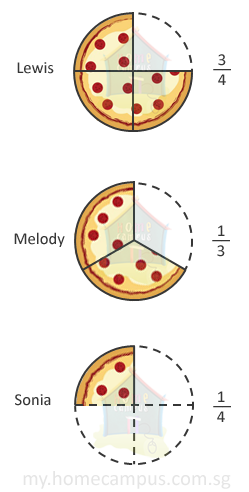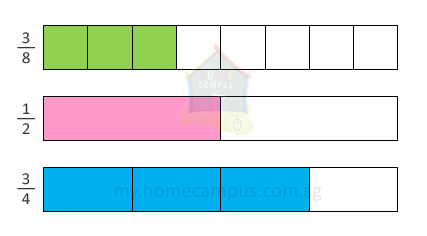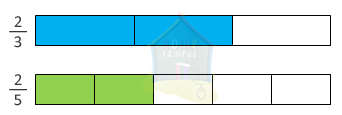## Comparing and Ordering Fractions

Practice Unlimited Questions

#### How to compare fractions?

Step 1: Express the fractions using a common denominator. For this you need to find out lowest common denominator.
Step 2: Create equivalent fractions by making the denominator common.
Step 3: The fraction with the smallest numerator is the smallest fraction while the fraction with the biggest numerator is the biggest fraction.

For better understanding follow the example below.

#### 1.   Lewis got 3 4 of a pizza.       Melody got 1 3 of a similar pizza.       Sonia got 1 4 of another similar pizza.a. Who got a bigger portion than Melody?
 3 4
is greater than
 1 3
.
Lewis got a bigger portion than Melody.

b. Who got a smaller portion than Melody?
 1 4
is smaller than
 1 3
.
Sonia got a smaller portion than Melody.

c. Who got the biggest portion?
 3 4
is greater than both
 1 3
and
 1 4
.
Lewis got the biggest portion.

d. Who got the smallest portion?
 1 4
is smaller than both
 3 4
and
 1 3
.
Sonia got the smallest portion.

#### 2. Which is greater: 2 9 or 1 3 ?

To compare the fractions, we must first express them using a common denominator.
So, we express
 1 3
as its equivalent fraction, which is
 3 9
.1 3
is greater than
 2 9
.

#### 3. Arrange the following fractions in order, beginning with the smallest. 1 2 , 3 4 , 3 8

Method 1:
Let's compare the fractions using fraction strips as shown below.Beginning with the smallest, the fractions should be arranged in the following order:
 3 8
,
 1 2
and
 3 4

Method 2:
To compare the fractions, we must first express them using a common denominator.
Fraction 1:
 1 2
=
 2 4
=
 4 8

Fraction 2:
 3 4
=
 6 8

Fraction 3:
 3 8

The fraction with the smallest numerator is the smallest fraction while the fraction with the biggest numerator is the biggest fraction.

So, beginning with the smallest, the fractions should be arranged in the following order:
 3 8
,
 1 2
and
 3 4

#### Tip:

When two or more fractions have the same numerator, example:
 2 3
and
 2 5
, it is easy to compare them.The greater fraction is the one with the smaller denominator.
Or,
The smaller fraction is the one with the greater denominator.